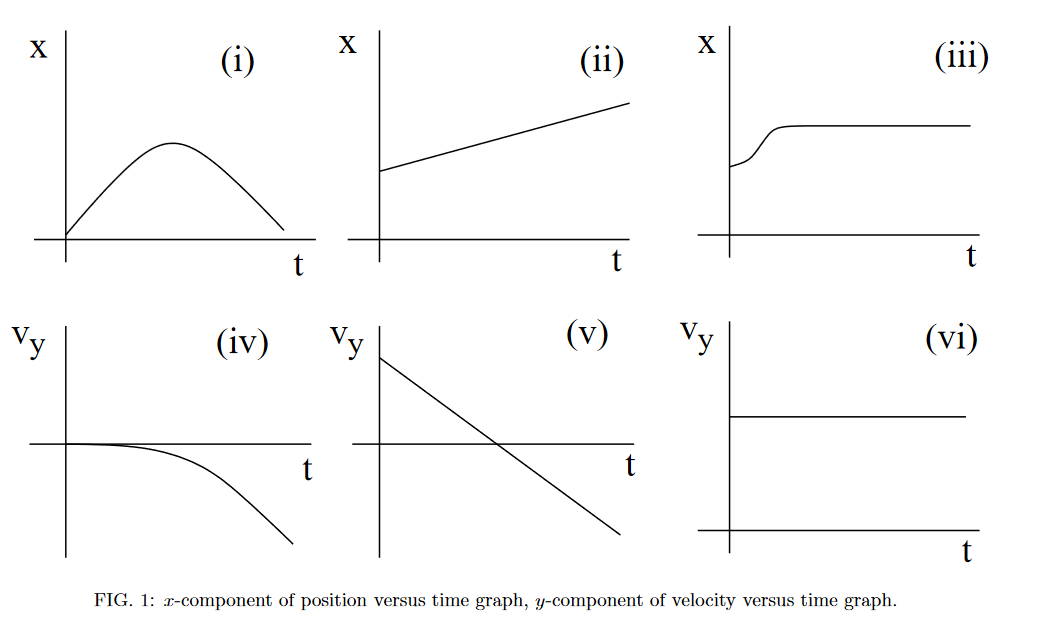# Graphs of Projectile Motion#

## Part 1#

Which of the below sets of graphs is consistent with projectile motion? Assume that gravity acts in the negative $$y$$-direction.##### Long Description of image: Figure of six graphs.
- Graph (i) is a displacement-time graph and it has a symmetric peak shape.
- Graph (ii) is a displacement-time graph. It is a straight line with a positive y-intercept.
- Graph (iii) is a displacement-time graph. It starts at a positive y-value and forms the right half of a trough (concave up and increasing) before forming a horizontal line.
- Graph (iv) is a velocity-time graph. It starts at the origin and then forms the right half of a peak (concave down and decreasing).
- Graph (v) is a velocity-time graph. It is a straight line with negative slope and positive y-intercept.
- Graph (vi) is a velocity-time graph. It is a horizontal line which crosses the vertical axis at a positive value.

Long description ends.

• Graph (i)

• Graph (ii)

• Graph (iii)

• Graph (iv)

• Graph (v)

• Graph (vi)